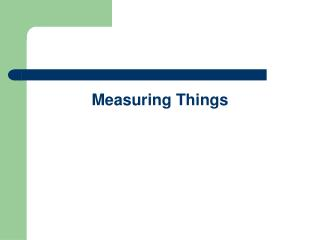# Measuring Things - PowerPoint PPT PresentationDownload PresentationMeasuring Things

Measuring ThingsDownload Presentation## Measuring Things

- - - - - - - - - - - - - - - - - - - - - - - - - - - E N D - - - - - - - - - - - - - - - - - - - - - - - - - - -
##### Presentation Transcript

1. Measuring Things

2. What are the 2 systems of measurement? • United States • Imperial system • Inches, feet, yards, pounds, ounces, quarts, etc. • Rest of the world • Metric System • Meters, liters, grams

3. In science we use the… • Metric system • Based on powers of 10 • Easy to convert between measurements • Based on the… • Meter • Liter • Gram

4. Question of the Day… What did King Henry drink?

5. King Henry drank ONE double chocolate milkshake

6. K H DaOD C M When converting, you must move the decimal point Meters -> Centimeters 1.00 meter = ? cm 1.00 meter = 100 cm

7. K H DaOD C M When converting, you must move the decimal point Kilometers -> Dekameters 5.50 kilometers = ? dekameters 5.50 kilometers = 550 dekameters

8. K H DaOD C M When converting, you must move the decimal point Millimeters -> Decimeters 153 millimeters = ? decimeters 153 millimeters = 1.53 decimeters

9. K H DaOD C M When converting, you must move the decimal point Centigrams -> Grams 42 centigrams -> ? grams 42 centigrams -> .42 grams

10. K H DaOD C M • Figure out the following… • 4.3 meters -> ? centimeters • 72 milliliters -> ? deciliters • 14.3 dekameters -> ? meters • 578.2 decigrams -> ? hectagrams • 6.22 liters -> ? milliliters

11. K H DaOD C M • Figure out the following… • 4.3 meters -> 430 centimeters • 72 milliliters -> 0.72 deciliters • 14.3 dekameters -> 143 meters • 578.2 decigrams -> 0.5782 hectagrams • 6.22 liters -> 6,220 milliliters